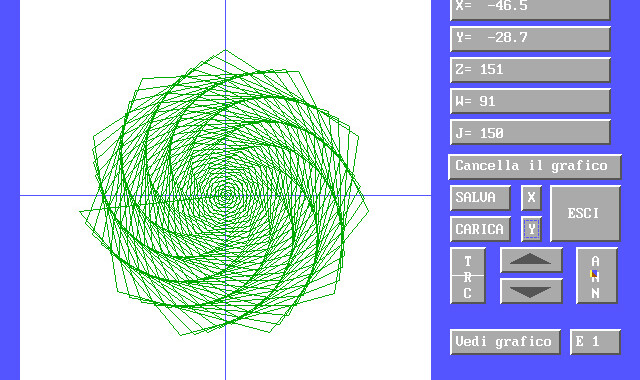# EQUATIO math graphing software

EQUATIO is a DOS math graphing software to trace 2D mathematical functions on a X-Y Cartesian plane. It is possible to write an expression for each Cartesian axis. The graph is traced point by point.

Available mathematical functions in EQUATIO math graphing software:

• ATN – arctangent of a number;
• ABS – absolute value of a number;
• COS – cosine of a number;
• SIN – sine of a number;
• SQR – square root of a number;
• TAN – tangent of a number;
• INT – integer value of a number;
• LOG – logarithm of a number;
• RND – random number;
• EXP – exponential of a number;
• SGN – sign of a number.

It is possible to set the color of the graphic, save and load all expressions and settings. You can also set variable minimum and maximum limits and graph zoom. Three variables with three different limits are available. An edge painting function is included, in order to paint a closed graph area.

Example of a single axis math function: Y = sin (X) + X.

Example of a two axes math function: X = W * cos (W); Y = W * sin (W) with multiple and discrete W values.

Programming language: Borland TurboBasic.
Project started on: Jan, 1993. : just for personal use.

### Ti piace questa pagina? Condividila:

Posted in Projects.